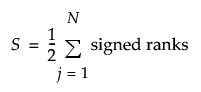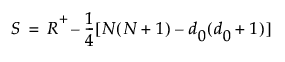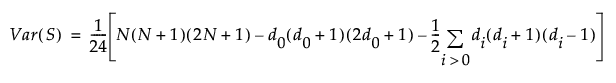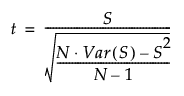Publication date: 11/29/2021

## Wilcoxon Signed Rank Test

The Wilcoxon signed-rank test can be used to test for the median of a single population or to test matched-pairs data for a common median. In the case of matched pairs, the test reduces to testing the single population of paired differences for a median of 0. The test assumes that the underlying population is symmetric.

The Wilcoxon test accommodates tied values. The test statistic is adjusted for differences of zero using a method suggested by Pratt. See Lehmann and D’Abrera (2006), Pratt (1959), and Cureton (1967).

#### Testing for the Median of a Single Population

There are N observations:

X1, X2,..., XN

The null hypothesis is:

H0: distribution of X is symmetric around m

The differences between observations and the hypothesized value m are calculated as follows:

Dj = Xj - m

#### Testing for the Equality of Two Population Medians with Matched Pairs Data

A special case of the Wilcoxon signed-rank test is applied to matched-pairs data.

There are N pairs of observations from two populations:

X1, X2,..., XN and Y1, Y2,..., YN

The null hypothesis is:

H0: distribution of X - Y is symmetric around 0

The differences between pairs of observations are calculated as follows:

Dj = Xj -Yj

#### Wilcoxon Signed-Rank Test Statistic

The test statistic is based on the sum of the signed ranks. Signed ranks are defined as follows:

The absolute values of the differences, Dj, are ranked from smallest to largest.

The ranks start with the value 1, even if there are differences of zero.

When there are tied absolute differences, they are assigned the average, or midrank, of the ranks of the observations.

Denote the rank or midrank for a difference Dj by Rj. Define the signed rank for Dj as follows:

If the difference Dj is positive, the signed rank is Rj.

If the difference Dj is zero, the signed rank is 0.

If the difference Dj is negative, the signed rank is -Rj.

The signed-rank statistic is computed as follows:Define the following:

d0 is the number of signed ranks that equal zero

R+ is the sum of the positive signed ranks

Then the following holds:#### Wilcoxon Signed-Rank Test P-Values

For N ≤ 20, exact p-values are calculated.

For N > 20, a Student’s t approximation to the statistic defined below is used. Note that a correction for ties is applied. See Iman (1974) and Lehmann and D’Abrera (2006).

Under the null hypothesis, the mean of S is zero. The variance of S is given by the following:The last summation in the expression for Var(S) is a correction for ties. The notation di for i > 0 represents the number of values in the ith group of nonzero signed ranks. (If there are no ties for a given signed rank, then di = 1 and the summand is 0.)

The statistic t given by the following has an approximate t distribution with N - 1 degrees of freedom: